# Integrals in Business Calculus Chapter Exam

Exam Instructions:

Choose your answers to the questions and click 'Next' to see the next set of questions. You can skip questions if you would like and come back to them later with the yellow "Go To First Skipped Question" button. When you have completed the practice exam, a green submit button will appear. Click it to see your results. Good luck!

### Page 1

#### Question 2 2. Evaluate the following.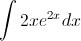#### Question 3 3. Evaluate the integral.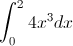#### Question 4 4. In the following equation, s is the _____.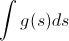#### Question 5 5. Which of the following graphs shows the correct region of integration for the integral below?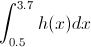### Page 2

#### Question 6 6. Evaluate the following.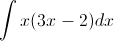#### Question 7 7. Evaluate the following integral.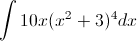#### Question 8 8. Evaluate the following.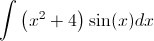#### Question 9 9. Which of the following is the BEST way to solve the integral below using integration by parts?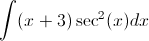#### Question 10 10. Which of the following integrals represents the area under the graph below?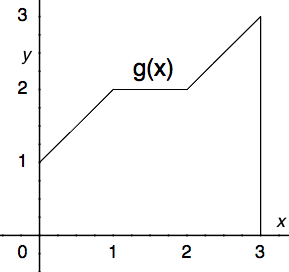### Page 3

#### Question 11 11. Use integration by parts to integrate the following: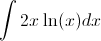#### Question 12 12. In the following equation, g(s) is the _____.#### Question 13 13. Use integration by part to integrate the following: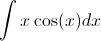#### Question 14 14. In the following equation, v(t) is the _____.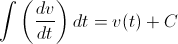#### Question 15 15. Calculate the integral.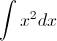### Page 4

#### Question 16 16. Given f(x) on the graph, what does z equal?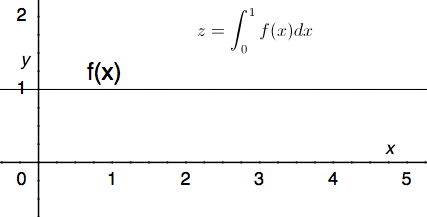#### Question 17 17. Evaluate the following integral.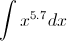#### Question 18 18. Evaluate the following integral.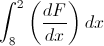#### Question 19 19. Evaluate the following integral.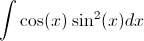#### Question 20 20. Which of the following expressions is equal to the integral below?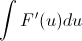### Page 5

#### Question 21 21. Assume the symbols are constants. Evaluate the following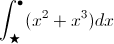#### Question 22 22. Use the fundamental theorem of calculus to evaluate the following integral.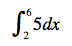#### Question 23 23. Integrate the following.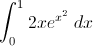#### Question 24 24. Given f(x) on the graph below, which of the following integrals will equal 0?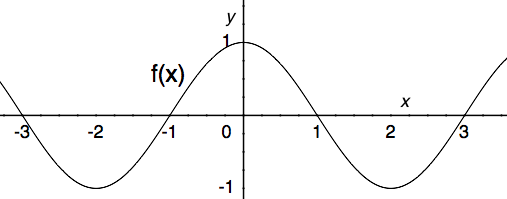#### Question 25 25. Integrate the following.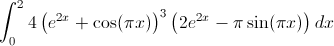### Page 6

#### Question 26 26. Which of the following shows the correct method for evaluating the integral below?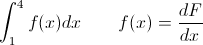#### Question 27 27. Evaluate the following integral.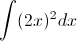#### Question 28 28. Evaluate the following integral.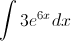#### Question 29 29. Which of the following can NOT be a solution for the integral below?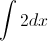#### Question 30 30. Evaluate the following integral.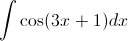#### Integrals in Business Calculus Chapter Exam Instructions

Choose your answers to the questions and click 'Next' to see the next set of questions. You can skip questions if you would like and come back to them later with the yellow "Go To First Skipped Question" button. When you have completed the practice exam, a green submit button will appear. Click it to see your results. Good luck!

Support